# Special Ordered Sets (SOS)

### Special Ordered Sets of Type One

A Special Ordered Set of type One (SOS1) is defined to be a set of variables for which not more than one member from the set may be non-zero in a feasible solution. All such sets are mutually exclusive of each other, the members are not subject to any other discrete conditions and are grouped together consecutively in the data.

The normal use of an SOS1 is to represent a set of mutually exclusive alternatives ordered in increasing values of size, cost or some other suitable units appropriate to the context of the model. This representation is a discrete programming extension of the separable programming model. There is a strong implied assumption that a non-linear function represented in this way is single valued over the range of its argument.

Consider a function g(y) represented by the points P1,...,PK as shown in figure 2.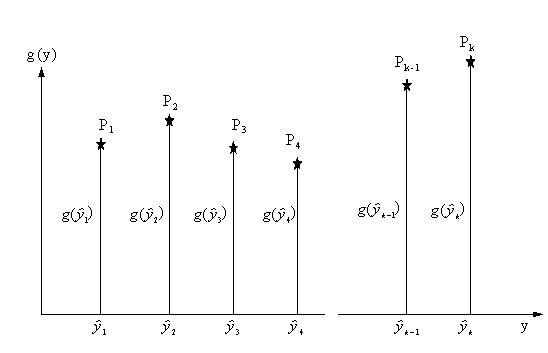Figure 2

Given the tabulated coordinatesk=1,...,K, the function g(y) may be represented as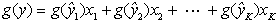(1)

where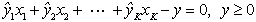(2)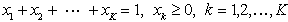(3)

The discrete function can take only one of the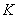possible values weighted by the variables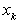, of which only one can be non-zero, and that must have the value one.

This requirement could be expressed by restricting each xk to be a binary variable but the alternative of defining them collectively as a special ordered set of type one, which is a direct statement of their nature, leads to a more efficient solution process.

The weighting variables xk are called special ordered set type one variables and the rows (1), (2), and (3) are called function rows, reference rows and convexity rows respectively. Should the SOS1's not represent a modelling of discrete, separable variables then none of these rows need actually exist, but there is an advantage to the system if it is aware of the reference rows at least.

### Special Ordered Sets of Type Two

A Special Ordered Set of type Two (SOS2) is a set of consecutive variables in which not more than two adjacent members may be non-zero in a feasible solution. All such sets are mutually exclusive of each other, the members are not subject to any other discrete conditions and each set is grouped together consecutively in the data.

SOS2s were introduced to make it easier to find global optimum solutions to problems containing piecewise linear approximations to a non-linear function of a single argument (as in classical Separable Programming). The overall problem has an otherwise LP or an IP structure except for such non-linear functions.

Consider the function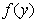illustrated in Figure 3 as a piecewise linear function in one variable defined over the closed intervals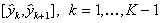, where the coordinates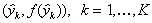, represent points P1,...,PK.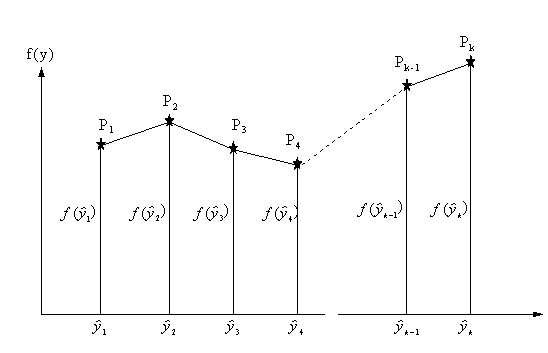Figure 3

Any point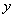in the closed interval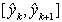may be written as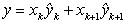where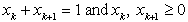.

Similarly, asis linear in the interval, it can be written as.

This leads to the representation ofusing a set of weighting variables,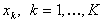, by the equality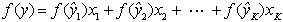(4)

where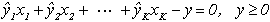(5).    (6)

Plus the added condition that not more than two adjacent variables can be non-zero at any one time.

The weighting variables xk are called the special ordered set type two variables and the rows (4), (5), and (6) are called function rows, reference rows and the convexity rows respectively, as in equations (1), (2) and (3) of Topic "Special Ordered Sets of Type One". Should the SOS2's not represent separable functions then none of these rows need actually exist, but there is an advantage to the system if it is aware of the reference rows at least.

The lp_solve implementation of SOS is the following:

A specially ordered set of degree N is a collection of variables where at most N variables may be non-zero. The non-zero variables must be contiguous (neighbours) sorted by the ascending value of their respective unique weights. In lp_solve, specially ordered sets may be of any cardinal type 1, 2, and higher, and may be overlapping. The number of variables in the set must be equal to, or exceed the cardinal SOS order.

lp_solve supports Special Ordered Sets via the API interface, in the MPS format and in the lp format (from release 4.0.1.11).

Below is a representation of a SOS in an MPS file, where each SOS is defined in its own SOS section, which should follow the BOUNDS section.

```0        1         2         3         4
1234567890123456789012345678901234567890
SOS
Sx CaseName  SOSName.  SOSpriority.
CaseName  VarName1  VarWeight1..
CaseName  VarName2  VarWeight2..

CaseName  VarNameN  VarWeightN..
```

x at the second line, position 3, defines is the order of the SOS. Due to limitations in the MPS format, N is restricted to the 1..9 range. Each SOS should be given a unique name, SOSName. lp_solve does not currently use case names for SOS'es and the CaseName could be any non-empty value.

Below is a representation of a SOS in an lp file.

```sos
SOS: VarName1: VarWeight1, VarName2: VarWeight2, ..., VarNameN: VarWeightN <= 1: SOSpriority;
```

In the lp format, the VarWeights are optional:

```sos
SOS: VarName1, VarName2, ..., VarNameN <= 1: SOSpriority;
```

In that case, the order of the variables define the VarWeights. So above is equal to:

```sos
SOS: VarName1: 1, VarName2: 2, ..., VarNameN: N <= 1: SOSpriority;
```

Also the SOSpriority is optional. In that case the order in which the SOS constraints are specified give them a priority.

The SOSpriority value determines the order in which multiple SOS'es are analysed in lp_solve.

VarWeight determines the adjacency of the variables. This is an importand piece of information. Remember that the SOS definition defines that the non-zero variables must be adjacent. The VarWeight values define this adjencency.

SOS1 Example:

```ROWS
L  c1
L  c2
N  COST
COLUMNS
x1        c1                 -1.   c2                  1.
x1        COST               -1.
x2        c1                 -1.   COST               -1.
x3        c1                  1.   c2                  1.
x3        COST               -3.
x4        c1                  1.   c2                 -3.
x4        COST               -2.
x5        COST               -2.
RHS
RHS       c1                 30.   c2                 30.
BOUNDS
UP COLBND    x1                 40.
UP COLBND    x2                  1.
UP COLBND    x5                  1.
SOS
S1 SOS       SOS                 1.
SOS       x1                  1.
SOS       x2                  2.
SOS       x3                  3.
SOS       x4                  4.
SOS       x5                  5.
ENDATA

In lp format:

min: -x1 -x2 -3 x3 -2 x4 -2 x5;
c1: -x1 -x2 +x3 +x4 <= 30;
c2: +x1 +x3 -3 x4 <= 30;
x1 <= 40;
x2 <= 1;
x5 <= 1;

sos
SOS: x1,x2,x3,x4,x5 <= 1;
```

The SOS definition says that either x1 or x2 or x3 or x4 or x5 may be taken. It also says that x1 is adjacent to x2 which is adjacent to x3 which is adjacent to x4 which is adjacent to x5.

The solution is:
```Value of objective function: -90

Actual values of the variables:
x1                   0
x2                   0
x3                   30
x4                   0
x5                   0
```

SOS2 Example:

```ROWS
L  c1
L  c2
N  COST
COLUMNS
x1        c1                 -1.   c2                  1.
x1        COST               -1.
x2        c1                 -1.   COST               -1.
x3        c1                  1.   c2                  1.
x3        COST               -3.
x4        c1                  1.   c2                 -3.
x4        COST               -2.
x5        COST               -2.
RHS
RHS       c1                 30.   c2                 30.
BOUNDS
UP COLBND    x1                 40.
UP COLBND    x2                  1.
UP COLBND    x5                  1.
SOS
S2 SOS       SOS                 1.
SOS       x1                  1.
SOS       x2                  2.
SOS       x3                  3.
SOS       x4                  4.
SOS       x5                  5.
ENDATA

In lp format:

min: -x1 -x2 -3 x3 -2 x4 -2 x5;
c1: -x1 -x2 +x3 +x4 <= 30;
c2: +x1 +x3 -3 x4 <= 30;
x1 <= 40;
x2 <= 1;
x5 <= 1;

sos
SOS: x1,x2,x3,x4,x5 <= 2;
```

The SOS definition says that two consecutive variables from x1, x2, x3, x4, x5 may be taken. It also says that x1 is adjacent to x2 which is adjacent to x3 which is adjacent to x4 which is adjacent to x5.

The solution is:
```Value of objective function: -91

Actual values of the variables:
x1                   0
x2                   1
x3                   30
x4                   0
x5                   0
```

SOS3 Example:

```ROWS
L  c1
L  c2
N  COST
COLUMNS
x1        c1                 -1.   c2                  1.
x1        COST               -1.
x2        c1                 -1.   COST               -1.
x3        c1                  1.   c2                  1.
x3        COST               -3.
x4        c1                  1.   c2                 -3.
x4        COST               -2.
x5        COST               -2.
RHS
RHS       c1                 30.   c2                 30.
BOUNDS
UP COLBND    x1                 40.
UP COLBND    x2                  1.
UP COLBND    x5                  1.
SOS
S3 SOS       SOS                 1.
SOS       x1                  1.
SOS       x2                  2.
SOS       x3                  3.
SOS       x4                  4.
SOS       x5                  5.
ENDATA

In lp format:

min: -x1 -x2 -3 x3 -2 x4 -2 x5;
c1: -x1 -x2 +x3 +x4 <= 30;
c2: +x1 +x3 -3 x4 <= 30;
x1 <= 40;
x2 <= 1;
x5 <= 1;

sos
SOS: x1,x2,x3,x4,x5 <= 3;
```

The SOS definition says that three consecutive variables from x1, x2, x3, x4, x5 may be taken. It also says that x1 is adjacent to x2 which is adjacent to x3 which is adjacent to x4 which is adjacent to x5.

The solution is:
```Value of objective function: -93.75

Actual values of the variables:
x1                   0
x2                   1
x3                   30.75
x4                   0.25
x5                   0
```

SOS4 Example:

```ROWS
L  c1
L  c2
N  COST
COLUMNS
x1        c1                 -1.   c2                  1.
x1        COST               -1.
x2        c1                 -1.   COST               -1.
x3        c1                  1.   c2                  1.
x3        COST               -3.
x4        c1                  1.   c2                 -3.
x4        COST               -2.
x5        COST               -2.
RHS
RHS       c1                 30.   c2                 30.
BOUNDS
UP COLBND    x1                 40.
UP COLBND    x2                  1.
UP COLBND    x5                  1.
SOS
S4 SOS       SOS                 1.
SOS       x1                  1.
SOS       x2                  2.
SOS       x3                  3.
SOS       x4                  4.
SOS       x5                  5.
ENDATA

In lp format:

min: -x1 -x2 -3 x3 -2 x4 -2 x5;
c1: -x1 -x2 +x3 +x4 <= 30;
c2: +x1 +x3 -3 x4 <= 30;
x1 <= 40;
x2 <= 1;
x5 <= 1;

sos
SOS: x1,x2,x3,x4,x5 <= 4;
```

The SOS definition says that four consecutive variables from x1, x2, x3, x4, x5 may be taken. It also says that x1 is adjacent to x2 which is adjacent to x3 which is adjacent to x4 which is adjacent to x5.

The solution is:
```Value of objective function: -233.75

Actual values of the variables:
x1                   40
x2                   1
x3                   50.75
x4                   20.25
x5                   0
```

SOS5 Example:

```ROWS
L  c1
L  c2
N  COST
COLUMNS
x1        c1                 -1.   c2                  1.
x1        COST               -1.
x2        c1                 -1.   COST               -1.
x3        c1                  1.   c2                  1.
x3        COST               -3.
x4        c1                  1.   c2                 -3.
x4        COST               -2.
x5        COST               -2.
RHS
RHS       c1                 30.   c2                 30.
BOUNDS
UP COLBND    x1                 40.
UP COLBND    x2                  1.
UP COLBND    x5                  1.
SOS
S5 SOS       SOS                 1.
SOS       x1                  1.
SOS       x2                  2.
SOS       x3                  3.
SOS       x4                  4.
SOS       x5                  5.
ENDATA

In lp format:

min: -x1 -x2 -3 x3 -2 x4 -2 x5;
c1: -x1 -x2 +x3 +x4 <= 30;
c2: +x1 +x3 -3 x4 <= 30;
x1 <= 40;
x2 <= 1;
x5 <= 1;

sos
SOS: x1,x2,x3,x4,x5 <= 5;
```

The SOS definition says that five consecutive variables from x1, x2, x3, x4, x5 may be taken. It also says that x1 is adjacent to x2 which is adjacent to x3 which is adjacent to x4 which is adjacent to x5.

The solution is:
```Value of objective function: -235.75

Actual values of the variables:
x1                   40
x2                   1
x3                   50.75
x4                   20.25
x5                   1
```